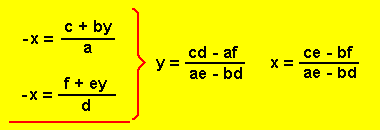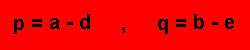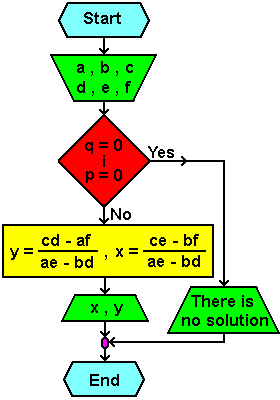# 6.1.2. System of linear equations1. phase
Solving systems of linear equations.

2. phase
Solve a system of two linear equations with two unknowns by equalization method of unknowns.

3. phase
The general form of the equation and the solution is:

ax + by + c = 0
dx + ey + f = 0
--------------------------------------------------
import parameters: a, b, c, d, e, fChecking parameters with expressions:4. phase
Flowchart:Citing of this page: Radic, Drago. " IT - Informatics Alphabet " Split-Croatia. {Date of access}. . Copyright © by Drago Radic. All rights reserved. | Disclaimer